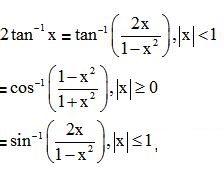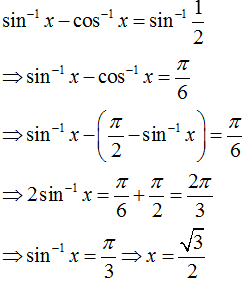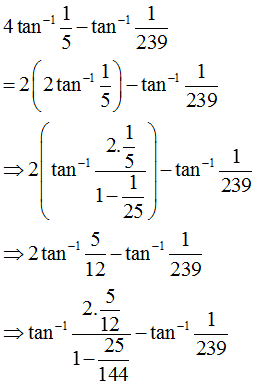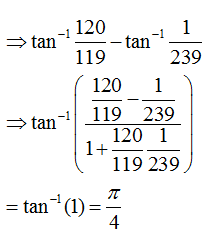# Topic: Inverse Trigonometric Functions (Test 1)

Topic: Inverse Trigonometric Functions
Q.1
is
A. non-negative.
B. negative
C. Zero
D. positive
Answer : Option B
Explaination / Solution:

there fore answer is negative
Workspace
Report
Q.2
The value of  is
A. 3+122
B. 3-122
C. 3+122
D. 3122
Answer : Option B
Explaination / Solution:

The value of  =

Workspace
Report
Q.3
If x then sin 2 θ is equal to
A. None of these.
B. 2x1+x2
C. 2x1x2
D. 1x21+x2
Answer : Option B
Explaination / Solution:

.

Workspace
Report
Q.4
is equal to
A. none of these.
B. −π/3
C. π/3
D. 2π/3
Answer : Option B
Explaination / Solution:

=.because ,
Workspace
Report
Q.5
holds true for all
A. |x|12
B. |x|1
C. none of these.
D. 0⩽x⩽1
Answer : Option D
Explaination / Solution:

This is true for all real values of x ∈[0,1].

Workspace
Report
Q.6
Which of the following is different from ?
A. sin1(2x1x2),|x|1
B. None of these
C. cos1(1x21+x2),|x|0
D.
Answer : Option B
Explaination / Solution:therefore , none of these is the right option.

Workspace
Report
Q.7
The number of solutions of the equation is
A. 2
B. infinite.
C. 3
D. 1
Answer : Option D
Explaination / Solution:hence there is only one solution

Workspace
Report
Q.8
4tan1(15)tan1(1239)=
A. π/3
B. π/2
C. π/4
D. π
Answer : Option C
Explaination / Solution:Workspace
Report
Q.9
The maximum value of sin x + cos x is
A. 1/√2.
B. 2
C. 1
D. √2
Answer : Option D
Explaination / Solution:

(12)+(12)=2
Workspace
Report
Q.10
When x=,x/2,then tan x, is
A. −∞
B. not defined.
C. 13
D. 1
Answer : Option B
Explaination / Solution:

tan(π2)=sin(π2)cos(π2)=10=notdefined
Workspace
Report